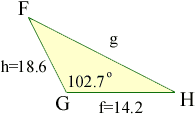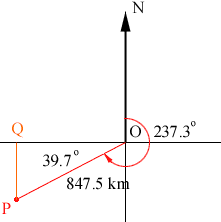Hi, Thanks for the help!! I have this ongoing trouble with trig and solving triangles with laws of cosines and sines!! For example if it asks to solve triangle FGH, given angle G=102.7 , side f=14.2, and h=18.6. Now do I use law of cosines because I don't have the measure of an angle and length of the opposite side??I don't know where to go from here,I am totally confused!!! I also have a problem with this word problem I have been doing. It asks: An airplane flies 847.5 km at a bearing of 237.3 degrees. How far south and west fo its original position is it? Huh? Please help! Thanks for your time Brett Student (doing gr11/12 math) Hi Brett, I drew a diagram of your triangle.Since you know the measure of the angle G, the form of the Law of Cosines to use is g2 = h2 + f2 - 2 h f Cos(G) For your second problem I drew a diagram with the initial position of the airplane at the origin O and north in the direction of the positive y-axis.Does this help? Penny Go to Math Central To return to the previous page use your browser's back button.